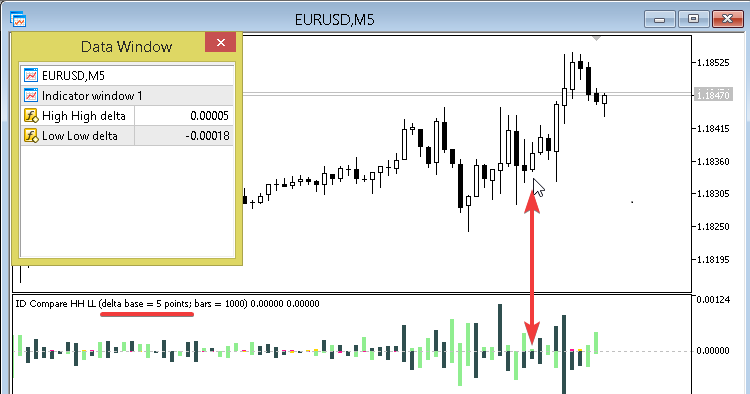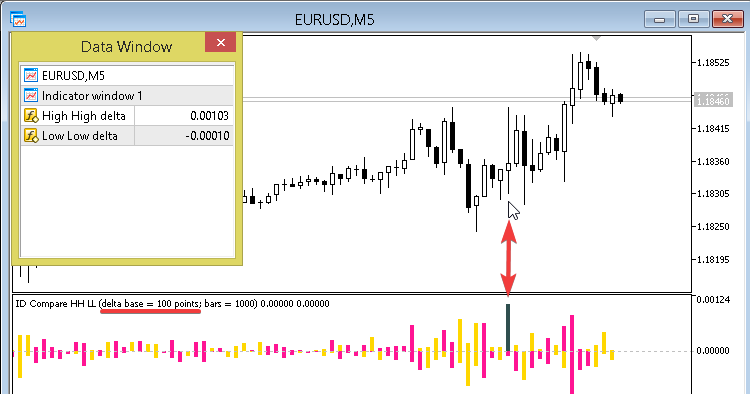# ID Compare HH LL and delta base – indicator for MetaTrader 5

• A+
category：MT5 INDICATORS

This oscillator shows in form of a histogram the differences in points between the successive:

• High of bars/candlesticks;
• Low of bars/candlesticks.

It also shows them in different colors, depending on:

• High and Low directions;
• amount of points set in the properties.### Features of histogram columns

• above the zero line shown in the separate window of this indicator are the differences between High[n] and High[n-1];
• below the zero line are the differences between Low[n] and Low[n-1];
• showing in different colors depending on the High and Low directions and on the sizes in points:
• For (High[n] < High[n-1]), as well as for (Low[n] < Low[n-1]):
• If the difference in points is greater than that set in the indicator properties, the default color = DarkSlateGray;
• If it is lower than that set in the properties, then the color = DeepPink.
• For (High[n] > High[n-1]), as well as for (Low[n] > Low[n-1]):
• If the absolute difference in points is greater than that set in the indicator properties, then the default color = LightGreen;
• If it is lower than that set in the properties, then the color = Gold.
• Histogram is plotted starting from the first bar following the zeroth bar.

### Input parameters

• i_delta_base - difference in points, for the values below which the following display colors are set by default: DeepPink and Gold;
• i_bars - the number of the last bars, for which the indicator values will be shown.